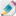# Arithmetic Sequences

##1, 5, 9, 13

These are sequences where the difference from one term to the next remains constant, like the one in the title! Arithmetic sequences crop up in all sorts of places and this unit looks at understanding how to generalise, sum and solve problems involving arithmetic sequences.

The following is a series of slides and videos that will help you understand, learn about and review this sub-topic.

Keep track of your progress and practice the exam questions on thisArithmetic Sequences record sheet

#### Slides Gallery

Use these slides to review the material and key points covered in the videos.

####What is an Arithmetic Sequence

This video describes the nature and properties of an arithmetic sequence.

###Finding the nth term of an arithmetic sequence

This video goes over the process of describing arithmetic sequences generally.

###Finding terms of an arithmetic sequence

How to find terms with a given position in the sequence. For example, for a given sequence, how do you find the 15th term?

###Finding the sum of an arithmetic sequence

How do we find the total of a given number of terms of an arithmetic sequence?

###Revise

This section of the page can be used for quick review. The flashcards help you go over key points and the quiz lets you practice answering questions on this subtopic.

#### Flash Cards

Review these condensed 'key point' flashcards to help you check and keep ideas fresh in your mind.

#### Quiz

Practice your understanding on these quiz questions. Check your answers when you are done and read the hints where you got stuck. If you find there are still some gaps in your understanding then go back to the videos and slides above.

###Exam Style Questions

The following questions are based on IB exam style questions from past exams. You should print these off (from the document at the top) and try to do these questions under exam conditions. Then you can check your work with the video solution.

.....